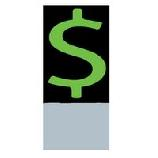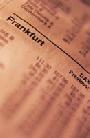Financial Terms Biased expectations theories

# Definition of Biased expectations theories## Biased expectations theories

Related: pure expectations theory.

# Related Terms:

## Pure expectations theory

A theory that asserts that the forward rates exclusively represent the expected
future rates. In other words, the entire term structure reflects the markets expectations of future short-term
rates. For example, an increasing sloping term structure implies increasing short-term interest rates. Related:
biased expectations theories

## Expectations hypothesis theories

theories of the term structure of interest rates which include the pure
expectations theory, the liquidity theory of the term structure, and the preferred habitat theory. These theories
hold that each forward rate equals the expected future interest rate for the relevant period. These three theories
differ, however, on whether other factors also affect forward rates, and how.
expectations theory of forward exchange rates A theory of foreign exchange rates that holds that the
expected future spot foreign exchange rate t periods in the future equals the current t-period forward exchange
rate.

## Homogenous expectations assumption

An assumption of Markowitz portfolio construction that investors
have the same expectations with respect to the inputs that are used to derive efficient portfolios: asset returns,
variances, and covariances.

## Local expectations theory

A form of the pure expectations theory which suggests that the returns on bonds
of different maturities will be the same over a short-term investment horizon.

## Rational expectations

The idea that people rationally anticipate the future and respond to what they see ahead.

## Return-to-maturity expectations

A variant of pure expectations theory which suggests that the return that an
investor will realize by rolling over short-term bonds to some investment horizon will be the same as holding
a zero-coupon bond with a maturity that is the same as that investment horizon.

## Unbiased predictor

A theory that spot prices at some future date will be equal to today's forward rates.## expectations theory of exchange rates

Theory that expected spot exchange rate equals the forward rate.

## Rational Expectations

The best forecasts that can be made given the data available and knowledge of how the economy operates. Rational expectations implies random errors, no systematic errors.